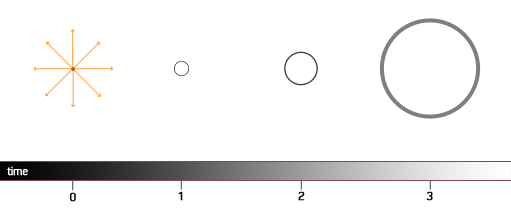# Point Source Energy Decay

When playing with explosions, I was trying to determine how much an explosion’s energy or force is lost as you move away from the source. After Googling a bit, I found out that as per Coulomb’s Law (which relates more to electric forces, but makes sense for pretty much any point energy source), the energy is inversely proportional to the square of the distance. Kudos to Matthew for helping me find that information.

I intuitively knew that this made sense, probably seen it in other places… but I couldn’t explain it. Why 1 / d²?

After thinking a bit more about it, I managed to pull off a simple yet formal proof to this for any point energy source like sound and light… and explosions!

### Circles on the water

You have to imagine an infinitely small, even null-sized energy source, like a point light in graphics programming. This point energy source emits energy in all directions equally. So let’s say it emits a single pulse at 0-time.This source emits a 3D sphere around it, which is very dense and concentrated at first (infinitely dense at t=0) but the same energy spreads and becomes less and less dense. This energy “density” defines how much actually hits a surface or object at any distance.

The energy “particles” (that doesn’t sound right, but it’s conceptual) that form that expanding ring are traveling away from the center at constant speed, we’re assuming no friction or resistance in the surrounding medium. It’s a vacuum.

We know that the area of a sphere’s surface is 4πr², where r is the radius of that sphere. Let’s calculate its area at different times, considering that it grows in radius linearly through time :

t0 : r = 0, a = 0
t1 : r = 1, a = 4π
t2 : r = 2, a = 16π
t3 : r = 3, a = 36π

ti : r = i, a = 4πi

So, now that we know the area, I ask the question : How much “energy density” is contained in one square unit of that sphere’s surface at any given time?

Simply put, that’s the inverse of its area. Since we know that the entire area contains all the initial pulse’s energy, one square unit divided by this entire area corresponds to the amount of energy it contains.

And we want to remove all units here, so let’s calculate the ratio to the size at t=1. That gives… :

t0 : a’ = +∞, ratio = +∞
t1 : a’ = 1/4π, ratio = 1
t2 : a’ = 1/16π, ratio = 1/4
t3 : a’ = 1/36π, ratio = 1/9

ti : a’ = 1/4πi, ratio = 1/i²

There you have it. The amount of energy at time t and an equal distance, is the inverse of the square of that value. That is to say… 1 / d²!

I rest my case. :D

## One thought on “Point Source Energy Decay”

1.Matthew Gallant says:

Yay physics! That’s a really good analogy.

This site uses Akismet to reduce spam. Learn how your comment data is processed.